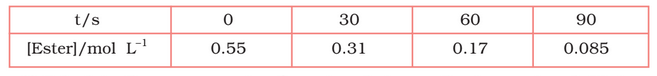Q

# In a pseudo first order reaction in water, the following results were obtained: Calculate the average rate of reaction between the time interval 30 to 60 seconds.

4.8 In pseudo first order hydrolysis of ester in water, the following results were obtained:(i) Calculate the average rate of reaction between the time interval 30 to 60 seconds.

Views

The average rate of reaction between the time 30 s to 60 s is expressed as-

Exams
Articles
Questions